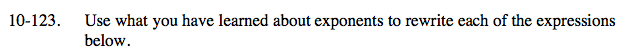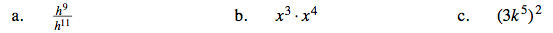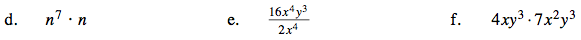### Home > CAAC > Chapter 10 > Lesson 10.4.1 > Problem10-123

10-123.Think about making ones and where any left over factors would remain.

$\frac{1}{h^2}$

How many factors of x are multiplied together?

x7

Write 3k5 twice and then follow the pattern from part (b).

(3k5)(3k5)Refer to part (b).

$\frac{16y^3}{2}=8y^3$

Refer to part (b).

28x3y6